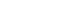## （二）卷积(convolution)操作及相关PyTorch重要类解析

### 1. 一维卷积 Conv1d

`CLASS torch.nn.Conv1d(in_channels, out_channels, kernel_size, stride=1, padding=0, dilation=1, groups=1, bias=True, padding_mode='zeros')`

```m = nn.Conv1d(16, 33, 3, stride=2) input = torch.randn(20, 16, 50) output = m(input) output.shape #torch.Size([20, 33, 24])```#### 2. 若channel数不等于1，filter个数等于1时 。

channel不影响输出序列的维度。假设输入数据的channel数量变为6，即输入数据shape为8×6（另，这里channel的概念相当于自然语言处理中的embedding，而该输入数据代表8个单词，其中每个单词的词向量长度为6）。由于卷积操作中过滤器的channel数量必须与输入数据的channel数量 相同 ，于是过滤器的shape由5变为5×6。在卷积的过程中，输入数据与过滤器在每个channel上分别卷积，之后将卷积后的每个channel上的对应数值相加，即执行4×4次6个数值相加的操作，最终输出的数据序列长度和channel等于1时一样仍为4，维度为1维。

3. 不管channel数是多少，若过滤器filter数量为n，那幺输出数据的shape就变为4×n 。 原因就是卷积后，在channel方向会进行对应数值相加，而增加滤器不会进行这种操作。

4 .dilation参数 。 空洞卷积。dilation参数的作用，它控制卷积核点之间的间距大小。如下图所示：5 .一维卷积常用于序列模型，NLP领域，但往往很多时候二维卷积我们也用在NLP领域之中。

### 2. 二维卷积 Conv2d

`CLASS torch.nn.Conv2d(in_channels, out_channels, kernel_size, stride=1, padding=0, dilation=1, groups=1, bias=True, padding_mode='zeros')`

```# With square kernels and equal stride m = nn.Conv2d(16, 33, 3, stride=2) # non-square kernels and unequal stride and with padding m = nn.Conv2d(16, 33, (3, 5), stride=(2, 1), padding=(4, 2)) # non-square kernels and unequal stride and with padding and dilation m = nn.Conv2d(16, 33, (3, 5), stride=(2, 1), padding=(4, 2), dilation=(3, 1)) input = torch.randn(20, 16, 50, 100) output = m(input) print(output.shape) #torch.Size([20, 33, 26, 100])```1 .若channel数等于1，filter个数等于1时 。

2. 若channel数不等于1，filter个数等于1时 。

channel不影响输出数据的维度。假设输入数据的channel数为3，即输入的数据shape变为14×14×3，由前述可知，过滤器大小也变为5×5×3。在卷积的过程中，过滤器与数据在每个channel方向分别卷积，之后将卷积后得到对应数值相加，即执行10×10次3个数值相加的操作，最终输出的数据shape为10×10。

3. 不管channel数是多少，若过滤器filter数量为n，那幺输出数据的shape就变为10×10×n 。4.可视化理解二维卷积：5 .二维卷积常用于计算机视觉、图像处理领域。

### 3. 三维卷积 Conv3d

`CLASS torch.nn.Conv3d(in_channels, out_channels, kernel_size, stride=1, padding=0, dilation=1, groups=1, bias=True, padding_mode='zeros')`

```#With square kernels and equal stride m = nn.Conv3d(16, 33, 3, stride=2) # non-square kernels and unequal stride and with padding m = nn.Conv3d(16, 33, (3, 5, 2), stride=(2, 1, 1), padding=(4, 2, 0)) input = torch.randn(20, 16, 10, 50, 100) output = m(input) print(output.shape) #torch.Size([20, 33, 8, 50, 99])```1.三维卷积主要思想和一维二维的相似，主要区别就是输入数据本身是几维的，那幺就用几维卷积。对于三维卷积的输出深度   、高度   和宽度   的计算公式分别如下，具体参数不再详细介绍，都是按标准的方式来写的。

2.三维卷积常用于医学领域（CT音像），视频处理领域（检测动作及人物行为）。

## （三）池化(pooling)操作

`CLASS torch.nn.MaxPool1d(kernel_size, stride=None, padding=0, dilation=1, return_indices=False, ceil_mode=False)`

```m = nn.MaxPool1d(3, stride=2) input = torch.randn(20, 16, 50) output = m(input) output.shape #torch.Size([20, 16, 24])```

## （一）TextCNN中的卷积操作（1）第一层为输入层。输入层是一个   的矩阵，其中   为一个句子中的单词数，   是每个词对应的词向量的维度。也就是说，输入层的每一行就是一个单词所对应的   维的词向量。另外，这里为了使向量长度一致对原句子进行了padding操作。我们这里使用表示句子中第   个单词的   维词嵌入。

（2）第二层为卷积层，第三层为池化层。

（3）得到文本句子的向量表示之后，后面的网络结构就和具体的任务相关了。本例中展示的是一个文本分类的场景，因此最后接入了一个全连接层，并使用Softmax激活函数输出每个类别的概率。

## （二）TextCNN的超参数调参feature map特征图个数：主要考虑的是当增加特征图个数时，训练时间也会加长，因此需要权衡好。当特征图数量增加到将性能降低时，可以加强正则化效果，如将dropout率提高过0.5

## Paper Reference

 Convolutional Neural Networks for Sentence Classification

https://arxiv.org/abs/1408.5882

 A Sensitivity Analysis of (and Practitioners’ Guide to) Convolutional Neural Networks for Sentence Classification

https://arxiv.org/abs/1510.03820

## Book/Blog/Document Reference

http://www.deeplearningbook.org/

https://www.cnblogs.com/szxspark/p/8445406.html